# Determine the force in each member of the roof truss shown. State whether each member is in tension or compression.

Question-AnswerCategory: Engineering MechanicsDetermine the force in each member of the roof truss shown. State whether each member is in tension or compression.

Determine the force in each member of the roof truss shown. State whether each member is in tension or compression.Step: 1

Draw the free body diagram of the entire truss.Step: 2

From the geometry of the figure,
From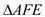From similar trianglesCalculate the angle of inclination.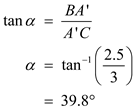Step: 3

From similar triangles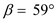Step: 4

Take moment about A as follows: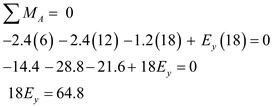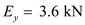Step: 5

Apply the equilibrium condition as follows: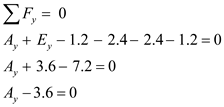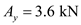Step: 6

Free body diagram of the joint A:Step: 7

Apply the equilibrium condition as follows: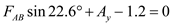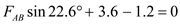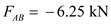Therefore, the force acting in the member AB is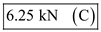Step: 8

Apply the equilibrium condition as follows: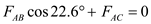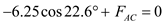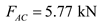Therefore, the force acting in the member AC is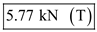.

Step: 9

Draw the free body diagram of the joint B: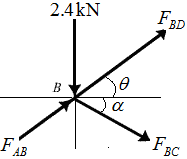Step: 10

Apply the equilibrium condition as follows: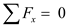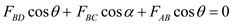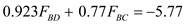…… (1)

Step: 11

Apply the equilibrium condition as follows: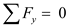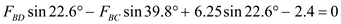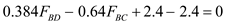Step: 12

Substituteforin equation (1).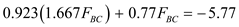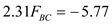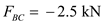Therefore, the force acting in the member BC is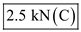.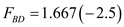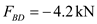Therefore, the force acting in the member BD is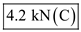.

Step: 13

Free body diagram of the joint C: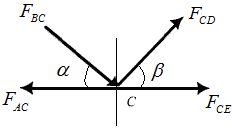Step: 14

Apply the equilibrium condition as follows: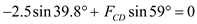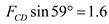Therefore, the force acting in the member CD is.

Step: 15

Apply the equilibrium condition as follows: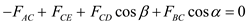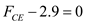Therefore, the force acting in the member CE is.

Step: 16

Draw the free body diagram of the joint D: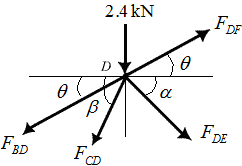Step: 17

Apply the equilibrium condition as follows: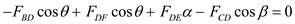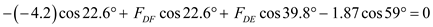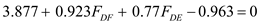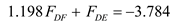…… (2)

Step: 18

Apply the equilibrium condition as follows: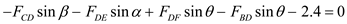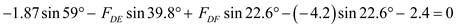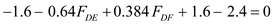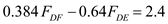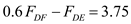…… (3)

Step: 19

Solve equations (2) and (3).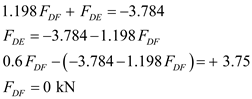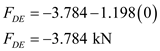Therefore, the force acting in the member DF is.
Therefore, the force acting in the member DE is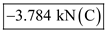.

Step: 20

Draw the free body diagram of the joint E:Step: 21

Apply the equilibrium condition as follows:Therefore, the force acting in the member EF is.